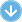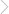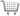88 Pages## Walker Maths 2.12 Probability Methods

### By Charlotte Walker, Victoria Walker | Copyright Year:2015 | ISBN-13: 9780170354240

Published:11/12/2014Walker Maths is a series of single standard workbooks containing high-quality, up to date material at NCEA Mathematics levels 1, 2 and 3.

The well-designed, write-on workbooks contain teaching material, including relevant formulae, and ample practice exercises along with sample tasks and questions. The workbooks reflect the content and style of the new standards, and allow teachers total flexibility in course design for students at all levels.

As a single standard series, Walker Maths offers Maths department the ability to buy titles all at once, or throughout the year as required.

A Walker Maths Digital Teacher Resource is available for \$9.95 per year for a single download. Each Digital Teacher Resource includes a Walker Maths eBook/projection file. Plus a selection of
• Worksheets
• Extra questions
• Teacher notes
• Videos
• Puzzle sheets
• Practice quizzes
• Worked solutions

### CONTENTS

Formula
Glossary
Probability revision
Graphs of distributions
Calculations from graphs of distributions
Describing graphs of distributions
The normal distribution
The standard normal distribution
Using Z to calculate a probability
Calculating a probability on the right side of the curve
Probabilities below a value
Probabilities from the right tail of the curve
Probabilities on the left of the curve
Probabilities from a tail on the left side of the curve
Probabilities from both sides of the curve
Probabilities from two tails of the curve
Calculating probabilities by difference
Using a graphics calculator to calculate probabilities
Mixing it up
Inverse normal calculations
Using a probability to find Z
Using Z to calculate the value of x — where Z is positive
Using Z to calculate the value of x — where Z is negative
Using a graphics calculator to calculate inverse probabilities
Using Z to calculate the mean
Using Z to calculate the standard deviation
Mixing it up
Mixed normal distribution problems
Probability trees
Probability tables
Tables of frequencies
Tables of probabilities
Risk
Absolute risk
Relative risk
Risk and tables

Charlotte Walker BEd, GradDipTchLn, MHealSc.Charlotte has over 10 years' experience in teaching Mathematics.

Victoria Walker BSc (Hons), GradDipTchLn. Victoria has twenty years' experience in teaching Mathematics. She has received a Jim Campbell award for teaching excellence (2003) and an Ernest Duncan award in Mathematics (2007).

### FEATURES

All workbooks are written for the current NCEA Achievement standards

New, fresh content – right up to date

Cost effective write-on workbooks for individual standards allows for flexibility in course design

Rigorous trialing of both text and answers

Clearly modeled worked examples

Plenty of practice opportunities

A high standard of design and production – the clean design allows for plenty of open space and text

Talented mother-daughter team with many years of combined teaching experience in New Zealand schools.